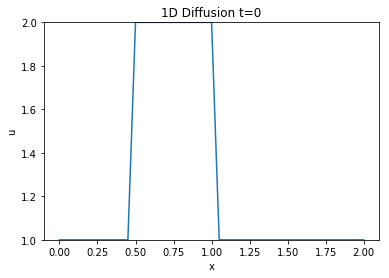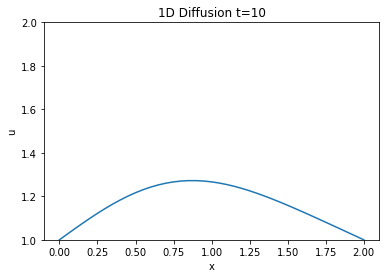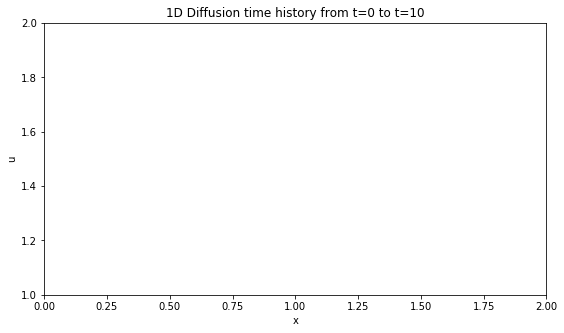# Step 3: Diffusion Equation in 1-d¶

Next, we tackle the one-dimensional diffusion equation:

$$\frac{\partial u}{\partial t} = \nu \frac{\partial^2 u}{\partial x^2}$$

First obvious difference is that — unlike our previous two simple equations — this equation has a second-order derivative. Before continuing we must learn how to discretize it.

## Discretizing $\frac{\partial^2 u}{\partial x^2}$¶

Any second order derivative can be understood geometrically as the line tangent to the curve given by a first derivative. To discretize this second order derivative we can use a Central difference scheme, a special combination of the two methods presented earlier Forward Difference and Backward Difference.

Consider the Taylor series expansions of $u_{i+1}$ and $u_{i-1}$ around $u_i$:

$$u_{i+1} = u_i + \Delta x \frac{\partial u}{\partial x}\biggr\rvert_i + \frac{\Delta x^2}{2} \frac{\partial^2 u}{\partial x^2}\biggr\rvert_i + \frac{\Delta x^3}{3!} \frac{\partial^3 u}{\partial x^3}\biggr\rvert_i + O(\Delta x^4)$$$$u_{i-1} = u_i - \Delta x \frac{\partial u}{\partial x}\biggr\rvert_i + \frac{\Delta x^2}{2} \frac{\partial^2 u}{\partial x^2}\biggr\rvert_i - \frac{\Delta x^3}{3!} \frac{\partial^3 u}{\partial x^3}\biggr\rvert_i + O(\Delta x^4)$$

If we add together both these expansions, the odd ordered derivative terms will cancel out. If we neglect any terms of $O(\Delta x^4)$ or higher because they are so small we can rearrange the sum of these two expansions to solve for our second-derivative.

$$u_{i+1} + u_{i-1} = 2u_i + \Delta x^2 \frac{\partial^2 u}{\partial x^2}\biggr\rvert_i$$$$\frac{\partial^2 u}{\partial x^2} = \frac{u_{i+1} - 2u_i + u_{i-1}}{\Delta x^2}$$

## Discretizing the full equation¶

We now have all the tools necessary to write the discretized version of the diffusion equation in 1D:

$$\frac{u^{n+1}_i - u^n_i}{\Delta t} = \nu \frac{u^{n}_{i+1} -2u^n_i + u^n_{i-1}}{\Delta x^2}$$

With only one unknown $u^{n+1}_i$ we can now rearrange this equation and obtain our final result.

$$u^{n+1}_i = u^n_i + \frac{\nu \Delta t}{\Delta x^2}(u^{n}_{i+1} - 2u^n_i + u^n_{i-1})$$

The above equation will now allow us to write a program to advance our solution in time and perform our simulation. As before, we need initial conditions, and we shall continue to use the one we obtained in the previous two steps.

## Libraries & Initial Conditions¶

In :
# Adding inline command to make plots appear under comments
import numpy as np
import matplotlib.pyplot as plt
import time, sys
%matplotlib inline

#Same initial conditions as in step 1 with courant number and viscosity added
grid_length = 2
grid_points = 41
dx = grid_length / (grid_points - 1)
nt = 500
nu = 0.3 # viscosity of the system
sig= 0.2 #courant number
dt = sig * dx**2 / nu #Dynamically scaling dt based on grid size to ensure convergence

#Initiallizing the shape of the wave to the same one from step 1 and displaying it
u = np.ones(grid_points)
u[int(.5/ dx):int(1 / dx + 1)] = 2
plt.plot(np.linspace(0,grid_length,grid_points), u);
plt.ylim(1,2);
plt.xlabel('x')
plt.ylabel('u')
plt.title('1D Diffusion t=0');## Applying the discretization¶

Now we apply the discretization as outlined above and check out the final results.

In :
un = np.ones(grid_points)

for n in range(nt): #Runs however many timesteps you set earlier
un = u.copy()   #copy the u array to not overwrite values
for i in range(1,grid_points-1):
u[i] = un[i] + nu * (dt/dx**2) * (un[i+1]- 2*un[i] + un[i-1])

In :
plt.plot(np.linspace(0,grid_length,grid_points), u);
plt.ylim(1,2);
plt.xlabel('x')
plt.ylabel('u')
plt.title('1D Diffusion t=10');### Results¶

Looks pretty unchanged, to have a better idea if it behaved the same way we will take a look at the animation.

## Animating the wave moving¶

In :
#Imports for animation and display within a jupyter notebook
from matplotlib import animation, rc
from IPython.display import HTML

#Generating the figure that will contain the animation
fig, ax = plt.subplots()
fig.set_size_inches(9, 5)
ax.set_xlim(( 0, grid_length))
ax.set_ylim((1, 2))
line, = ax.plot([], [], lw=2)
plt.xlabel('x')
plt.ylabel('u')
plt.title('1D Diffusion time history from t=0 to t=10');
#Resetting the U wave back to initial conditions
u = np.ones(grid_points)
u[int(.5/ dx):int(1 / dx + 1)] = 2In :
#Initialization function for funcanimation
def init():
line.set_data([], [])
return (line,)

In :
#Main animation function, each frame represents a time step in our calculation
def animate(j):
x = np.linspace(0, grid_length, grid_points)
un = u.copy()   #copy the u array to not overwrite values
for i in range(1,grid_points-1):
u[i] = un[i] + nu * (dt/dx**2) * (un[i+1]- 2*un[i] + un[i-1])
line.set_data(x, u)
return (line,)

In :
anim = animation.FuncAnimation(fig, animate, init_func=init,
frames=nt, interval=20)

#anim.save('../gifs/1dDiff.gif',writer='imagemagick',fps=60)
HTML(anim.to_jshtml())


## Conclusion¶

This cool PDE simulates the behaviour of a quantity —a gas, energy, temperature etc—diffusing unifformly through an environment. It's evolution over a large period of time shows how it reaches an equilibrium where most of the values are spread out.

Next we will take a look at the Burgers' equation with a new initial condition and establish some boundary condiitons.